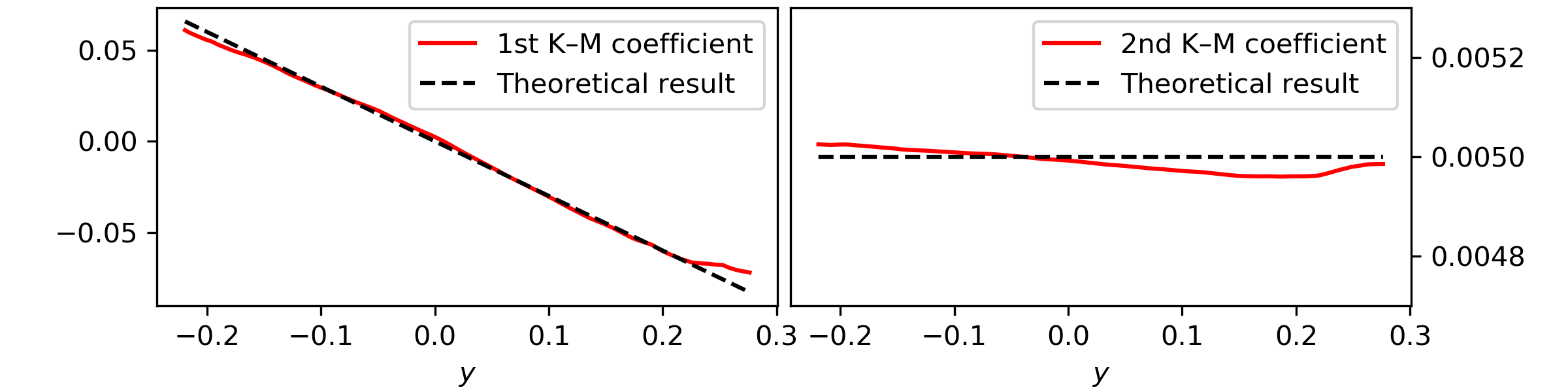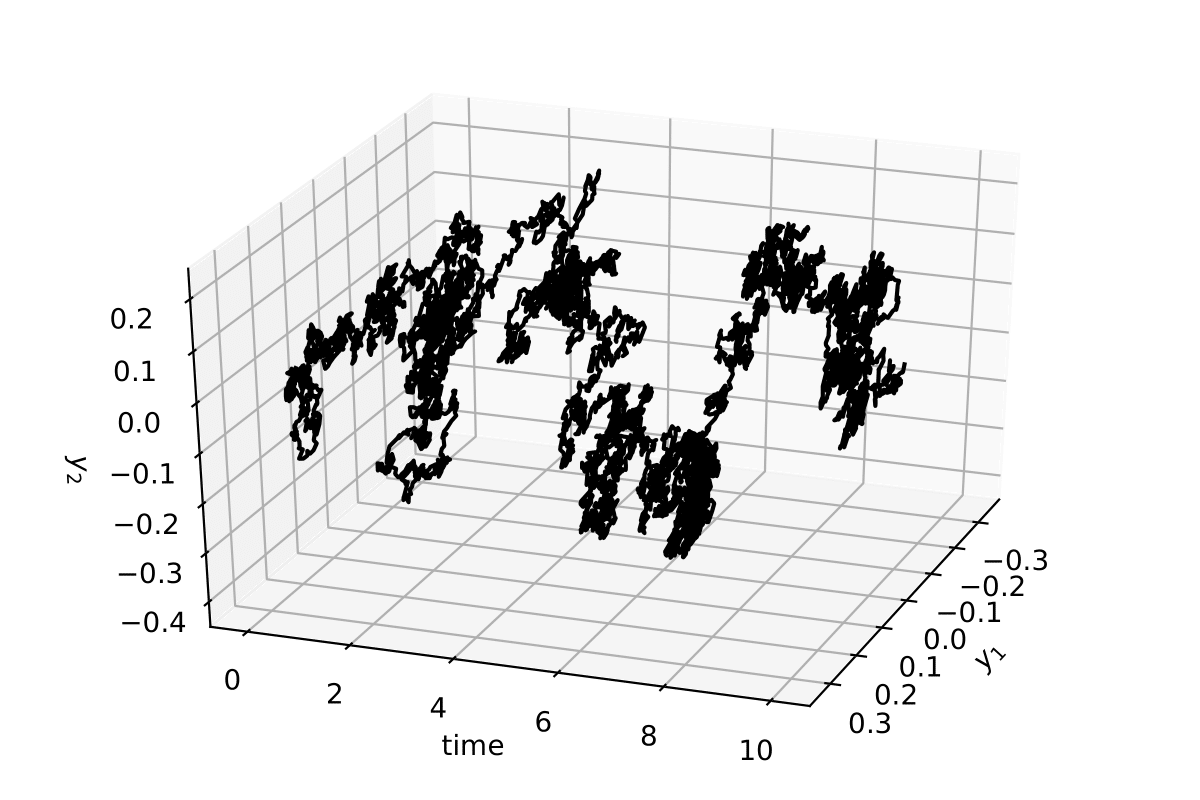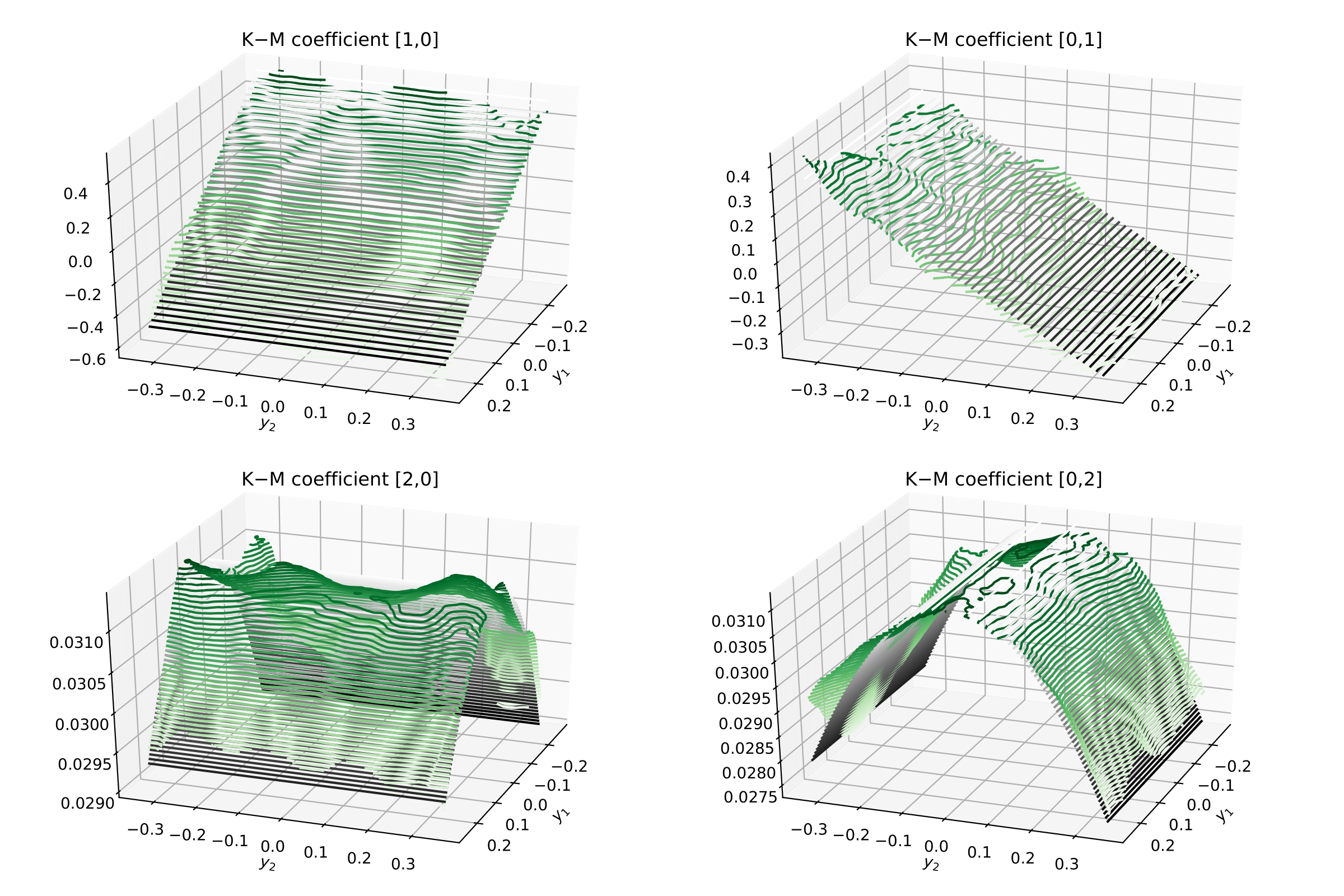# Kramers—Moyal¶

kramersmoyal is a python package designed to obtain the Kramers—Moyal coefficients, or conditional moments, from stochastic data of any dimension. It employs kernel density estimations, instead of a histogram approach, to ensure better results for low number of points as well as allowing better fitting of the results.

# Installation¶

For the moment the library is available from TestPyPI, so you can use

pip install -i https://test.pypi.org/simple/ kramersmoyal


Then on your favourite editor just use

from kramersmoyal import km


From here you can simply call

import numpy as np

# Number of bins
bins = np.array()

# Choose powers to calculate
powers = np.array([, ])

# And here x is your (1D, 2D, 3D) data
kmc, edge = km(x, bins = bins, powers = powers)


The library depends on numpy and scipy.

# A one-dimensional stochastic process¶

## The theory¶

Take, for example, the well-documented one-dimension Ornstein—Uhlenbeck process, also known as Vašíček process. This process is governed by two main parameters: the mean-reverting parameter $$\theta$$ and the diffusion or volatility coefficient $$\sigma$$

$\mathrm{d}y(t) = -\theta y(t)\mathrm{d}t + \sigma \mathrm{d}W(t)$

which can be solved in various ways. For our purposes, recall that the drift coefficient, i.e., the first-order Kramers—Moyal coefficient, is given by $$\mathcal{M}^{}(y) = -\theta y$$ and the second-order Kramers—Moyal coefficient is $$\mathcal{M}^{}(y) = \sigma^2 / 2$$, i.e., the diffusion.

For this example let’s take $$\theta=0.3$$ and $$\sigma=0.1$$, over a total time of 500 units, with a sampling of 1000 Hertz, and from the generated data series retrieve the two parameters, the drift $$-\theta y(t)$$ and diffusion $$\sigma$$.

## Integrating an Ornstein—Uhlenbeck process¶

Here is a short code on generating a Ornstein—Uhlenbeck stochastic trajectory with a simple Euler–Maruyama integration method

# integration time and time sampling
t_final = 500
delta_t = 0.001

# The parameters theta and sigma
theta = 0.3
sigma = 0.1

# The time array of the trajectory
time = np.arange(0, t_final, delta_t)

# Initialise the array y
y = np.zeros(time.size)

# Generate a Wiener process
dw = np.random.normal(loc = 0, scale = np.sqrt(delta_t), size = time.size)

# Integrate the process
for i in range(1,time.size):
y[i] = y[i-1] - theta*y[i-1]*delta_t + sigma*dw[i]


From here we have a plain example of an Ornstein—Uhlenbeck process, always drifting back to zero, due to the mean-reverting drift $$-\theta y(t)$$. The effect of the noise can be seen across the whole trajectory.## Using kramersmoyal¶

Take the timeseries $$y(t)$$ and let’s study the Kramers—Moyal coefficients. For this let’s look at the drift and diffusion coefficients of the process, i.e., the first and second Kramers—Moyal coefficients, with an epanechnikov kernel

# Choose number of points of you target space
bins = np.array()

# Choose powers to calculate
powers = np.array([, ])

bw = 0.15

# The kmc holds the results, where edges holds the binning space
kmc, edges = km(y, kernel = kernels.epanechnikov, bw = bw, bins = bins, powers = powers)


This results inNotice here that to obtain the Kramers—Moyal coefficients you need to multiply kmc by the timestep delta_t. This normalisation stems from the Taylor-like approximation, i.e., the Kramers—Moyal expansion ($$\delta t \to 0$$).

# A two-dimensional diffusion process¶

## Theory¶

A two-dimensional diffusion process is a stochastic process that comprises two $$W(t)$$ and allows for a mixing of these noise terms across its two dimensions.

$\begin{split}\begin{pmatrix} \mathrm{d}y_1(t) \\ \mathrm{d}y_2(t) \end{pmatrix}= \begin{pmatrix} N_1(y) \\ N_2(y) \end{pmatrix} \mathrm{d} t + \begin{pmatrix} g_{1,1}(y) & g_{1,2}(y) \\ g_{2,1}(y) & g_{2,2}(y) \end{pmatrix} \begin{pmatrix} \mathrm{d}W_1 \\ \mathrm{d}W_2 \end{pmatrix}\end{split}$

with $$N$$ the drift vector and $$g$$ the diffusion matrix, which can be state dependent. We define, as the previous example, a process identical to the Ornstein—Uhlenbeck process, with

$\begin{split}N = \begin{pmatrix} - N_1 y_1 \\ - N_2 y_2 \end{pmatrix}\end{split}$

and we take $$N_1=2.0$$ and $$N_2=1.0$$. For this particular case a more involved diffusion matrix $$g$$ will be used. Let the matrix $$g$$ be state-dependent, i.e., dependent of the actual values of $$y_1$$ and $$y_2$$ via

$\begin{split}g = \begin{pmatrix} \frac{g_{1,1} }{1+e^{y_1^2}} & g_{1,2} \\ g_{2,1} & \frac{g_{2,2} }{1+e^{y_2^2}} \end{pmatrix}\end{split}$

and we will take $$g_{1,1} = g_{2,2}=0.5$$ and $$g_{1,2} = g_{2,1} = 0$$.

## Integrating a 2-dimensional process¶

Taking the above parameters and writing again an Euler–Maruyama integration method

# integration time and time sampling
t_final = 2000
delta_t = 0.001

# Define the drift vector N
N = np.array([2.0, 1.0])

# Define the diffusion matrix g
g = np.array([[0.5, 0.0], [0.0, 0.5]])

# The time array of the trajectory
time = np.arange(0, t_final, delta_t)

# Initialise the array y
y = np.zeros([time.size, 2])

# Generate two Wiener processes with a scale of np.sqrt(delta_t)
dW = np.random.normal(loc = 0, scale = np.sqrt(delta_t), size = [time.size, 2])

# Integrate the process (takes about 20 secs)
for i in range(1, time.size):
y[i,0] = y[i-1,0]  -  N * y[i-1,0] * delta_t + g[0,0]/(1 + np.exp(y[i-1,0]**2)) * dW[i,0]  +  g[0,1] * dW[i,1]
y[i,1] = y[i-1,1]  -  N * y[i-1,1] * delta_t + g[1,0] * dW[i,0]  +  g[1,1]/(1 + np.exp(y[i-1,1]**2)) * dW[i,1]


The stochastic trajectory in 2 dimensions for 10 time units (10000 data points)## Back to kramersmoyal and the Kramers—Moyal coefficients¶

First notice that all the results now will be two-dimensional surfaces, so we will need to plot them as such

# Choose the size of your target space in two dimensions
bins = np.array([300, 300])

# Introduce the desired orders to calculate, but in 2 dimensions
powers = np.array([[0,0], [1,0], [0,1], [1,1], [2,0], [0,2], [2,2]])
# insert into kmc:   0      1      2      3      4      5      6

# Notice that the first entry in [,] is for the first dimension, the
# second for the second dimension...

# Choose a desired bandwidth bw
bw = 0.1

# Calculate the Kramers−Moyal coefficients
kmc, edges = km(y, bw = bw, bins = bins, powers = powers)

# The K−M coefficients are stacked along the first dim of the
# kmc array, so kmc[1,...] is the first K−M coefficient, kmc[2,...]
# is the second. These will be 2-dimensional matrices.


Now one can visualise the Kramers–Moyal coefficients (surfaces) in green and the respective theoretical surfaces in black. (Don’t forget to normalise: kmc * delta_t).